# 数据结构与算法(4)——优先队列和堆# 什么是优先队列？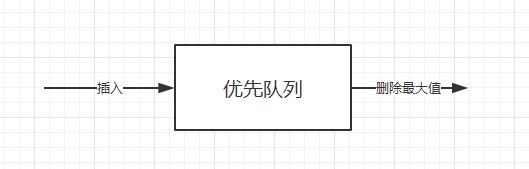1.优先队列的主要操作

• insert(key, data)：插入键值为key的数据到优先队列中，元素以其key进行排序；
• deleteMin/deleteMax：删除并返回最小/最大键值的元素；
• getMinimum/getMaximum：返回最小/最大剑指的元素，但不删除它；

2.优先队列的辅助操作

• 第k最小/第k最大：返回优先队列中键值为第k个最小/最大的元素；
• 大小（size）：返回优先队列中的元素个数；
• 堆排序（Heap Sort）：基于键值的优先级将优先队列中的元素进行排序；

## 优先队列的应用

• 数据压缩：赫夫曼编码算法；
• 最短路径算法：Dijkstra算法；
• 最小生成树算法：Prim算法；
• 事件驱动仿真：顾客排队算法；
• 选择问题：查找第k个最小元素；
• 等等等等….

# 堆和二叉堆

## 什么是堆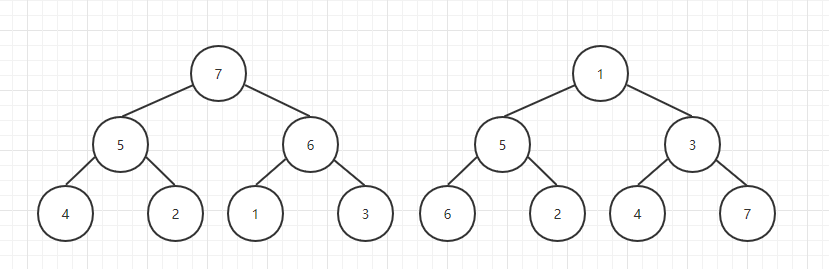## 二叉堆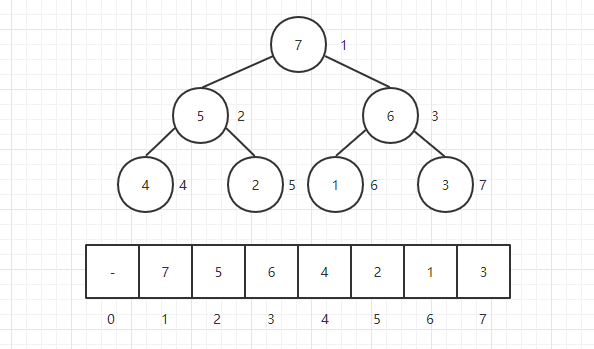• 每个结点的左孩子为下标i的2倍：left child(i) = i * 2；每个结点的右孩子为下标i的2倍加1：right child(i) = i * 2 + 1
• 每个结点的父亲结点为下标的二分之一：parent(i) = i / 2，注意这里是整数除，2和3除以2都为1，大家可以验证一下；
• 注意：这里是把下标为0的地方空出来了的，主要是为了方便理解，如果0不空出来只需要在计算的时候把i值往右偏移一个位置就行了（也就是加1，大家可以试试，下面的演示也采取这样的方式）；

### 二叉堆的相关操作

#### 堆的基本结构

public class MaxHeap<E extends Comparable<E>> {
private Array<E> data;
public MaxHeap(int capacity){ data = new Array<>(capacity); }
public MaxHeap(){ data = new Array<>(); }
// 返回堆中的元素个数
public int size(){ return data.getSize(); }
// 返回一个布尔值, 表示堆中是否为空
public boolean isEmpty(){ return data.isEmpty(); }
// 返回完全二叉树的数组表示中，一个索引所表示的元素的父亲节点的索引
private int parent(int index){
if(index == 0)
throw new IllegalArgumentException("index-0 doesn't have parent.");
return (index - 1) / 2;
}
// 返回完全二叉树的数组表示中，一个索引所表示的元素的左孩子节点的索引
private int leftChild(int index){ return index * 2 + 1; }
// 返回完全二叉树的数组表示中，一个索引所表示的元素的右孩子节点的索引
private int rightChild(int index){ return index * 2 + 2; }
}

#### 向堆中添加元素和Sift Up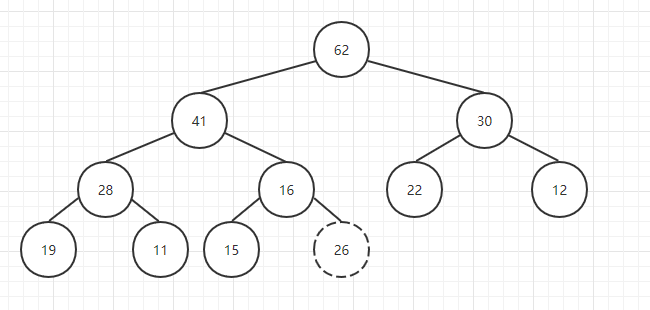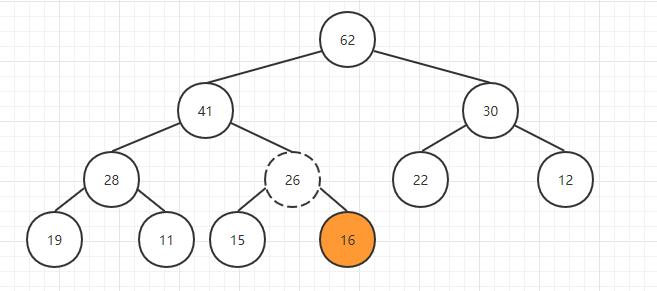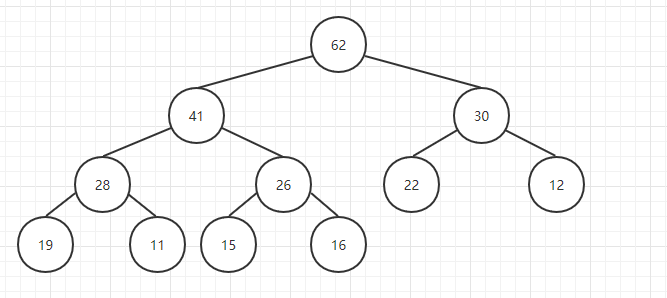// 向堆中添加元素
siftUp(data.getSize() - 1);
}

private void siftUp(int k){

while(k > 0 && data.get(parent(k)).compareTo(data.get(k)) < 0 ){
data.swap(k, parent(k));
k = parent(k);
}
}

#### 取出堆中的最大元素和Sift Down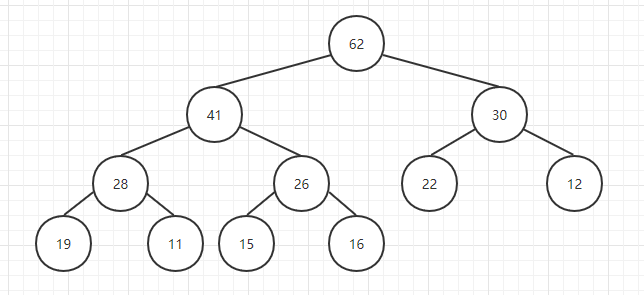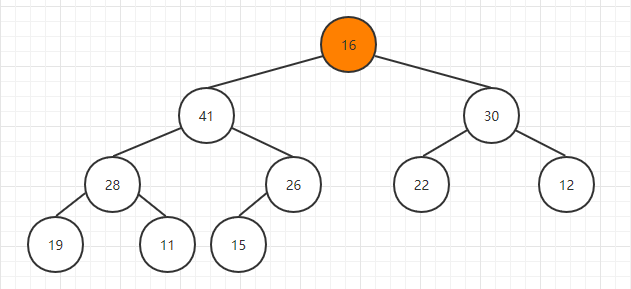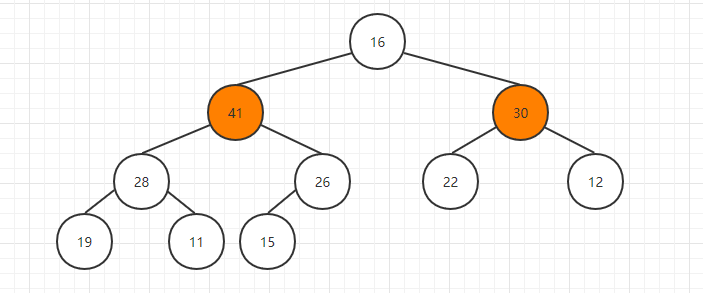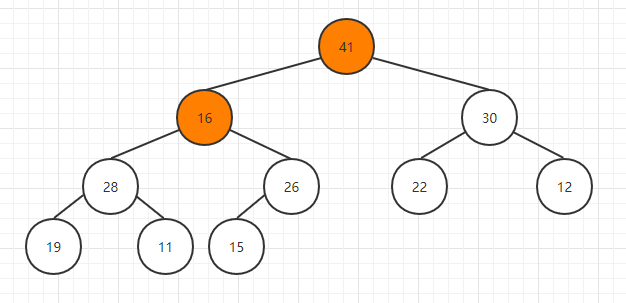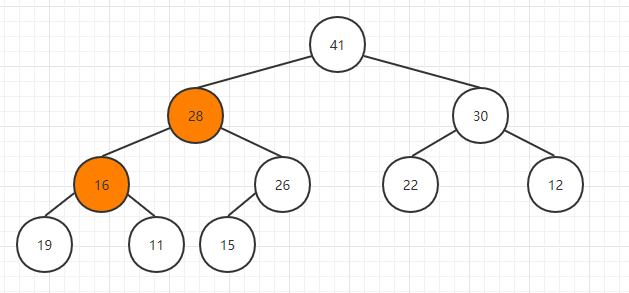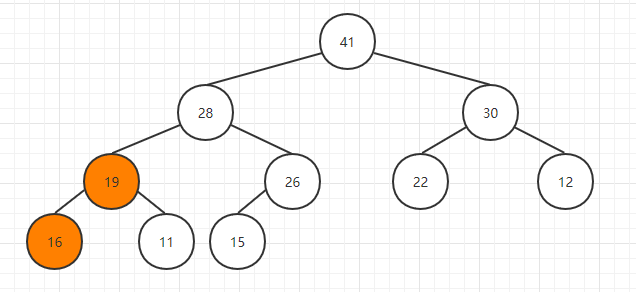// 看堆中的最大元素
public E findMax(){
if(data.getSize() == 0)
throw new IllegalArgumentException("Can not findMax when heap is empty.");
return data.get(0);
}

// 取出堆中最大元素
public E extractMax(){

E ret = findMax();

data.swap(0, data.getSize() - 1);
data.removeLast();
siftDown(0);

return ret;
}

private void siftDown(int k){

while(leftChild(k) < data.getSize()){
int j = leftChild(k); // 在此轮循环中,data[k]和data[j]交换位置
if( j + 1 < data.getSize() &&
data.get(j + 1).compareTo(data.get(j)) > 0 )
j ++;
// data[j] 是 leftChild 和 rightChild 中的最大值

if(data.get(k).compareTo(data.get(j)) >= 0 )
break;

data.swap(k, j);
k = j;
}
}

#### Replace 和 Heapify

Replace这个操作其实就是取出堆中最大的元素之后再新插入一个元素，常规的做法是取出最大元素之后，再利用上面的插入新元素的操作对堆进行Sift Up操作，但是这里有一个小技巧就是直接使用新元素替换掉堆顶元素，之后再进行Sift Down操作，这样就把两次O(logn）的操作变成了一次O(logn)：

// 取出堆中的最大元素，并且替换成元素e
public E replace(E e){

E ret = findMax();
data.set(0, e);
siftDown(0);
return ret;
}

Heapify翻译过来就是堆化的意思，就是将任意数组整理成堆的形状，通常的做法是遍历数组从0开始添加创建一个新的堆，但是这里存在一个小技巧就是把当前数组就看做是一个完全二叉树，然后从最后一个非叶子结点开始进行Sift Down操作就可以了，最后一个非叶子结点也很好找，就是最后一个结点的父亲结点，大家可以验证一下：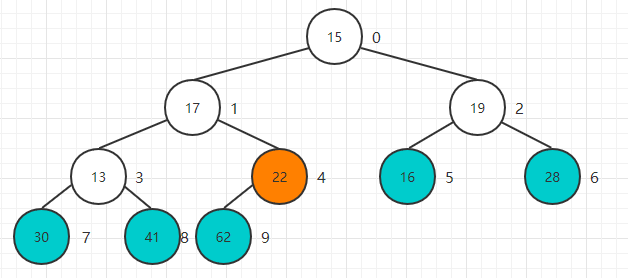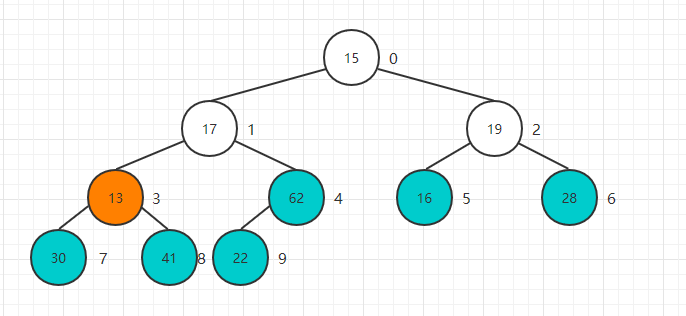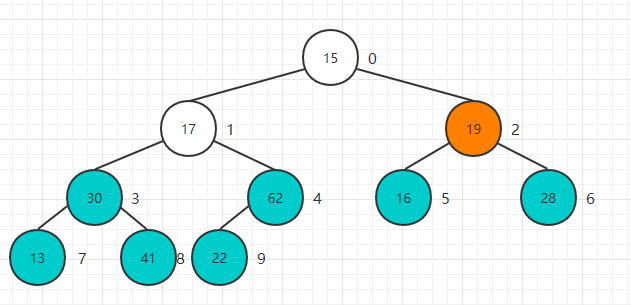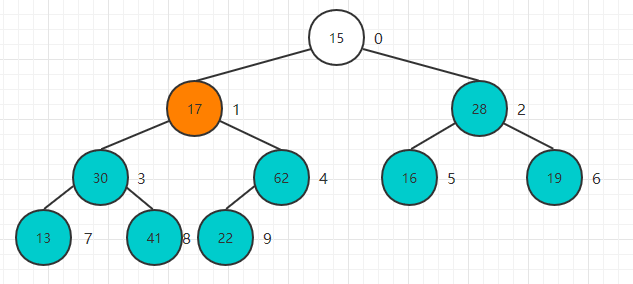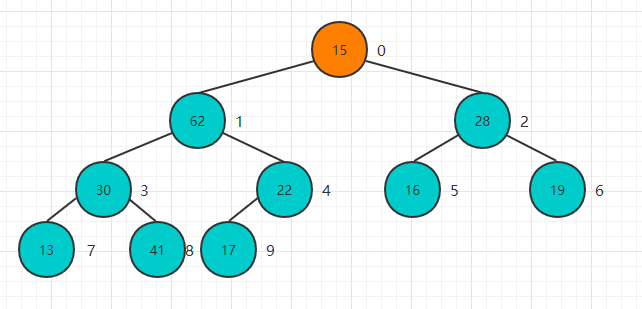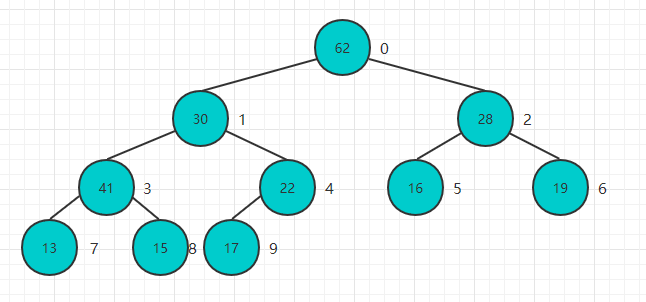public MaxHeap(E[] arr){
data = new Array<>(arr);
for(int i = parent(arr.length - 1) ; i >= 0 ; i --)
siftDown(i);
}

## 基于堆的优先队列

public class PriorityQueue<E extends Comparable<E>> implements Queue<E> {

private MaxHeap<E> maxHeap;

public PriorityQueue(){ maxHeap = new MaxHeap<>(); }
@Override
public int getSize(){ return maxHeap.size(); }
@Override
public boolean isEmpty(){ return maxHeap.isEmpty(); }
@Override
public E getFront(){ return maxHeap.findMax(); }
@Override
public void enqueue(E e){ maxHeap.add(e); }
@Override
public E dequeue(){ return maxHeap.extractMax(); }
}

## Java中的PriorityQueue

// 默认为最小堆
PriorityQueue<Integer> pq = new PriorityQueue<>();

while (!pq.isEmpty()) {
System.out.println(pq.poll() + ", ");
}
System.out.println();
System.out.println("————————————————————————");

// 使用Lambda表达式传入自己的比较器转换成最大堆
PriorityQueue<Integer> pq2 = new PriorityQueue<>((a, b) -> b - a);

while (!pq2.isEmpty()) {
System.out.println(pq2.poll() + ", ");
}

# LeetCode相关题目整理

## 23. 合并K个排序链表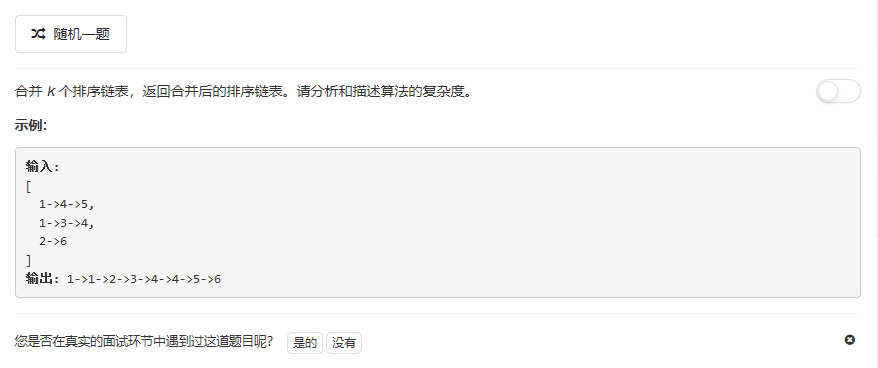public ListNode mergeKLists(ListNode[] lists) {
if (lists == null || lists.length == 0) return null;

PriorityQueue<ListNode> q = new PriorityQueue<>(Comparator.comparing(node -> node.val));
for (int i = 0; i < lists.length; i++) {
if (lists[i] != null) {
}
}

ListNode dummy = new ListNode(0);
ListNode tail = dummy;

while (!q.isEmpty()) {
tail.next = q.poll();
tail = tail.next;
if (tail.next != null) {
}
}

return dummy.next;
}

## 215. 数组中的第K个最大元素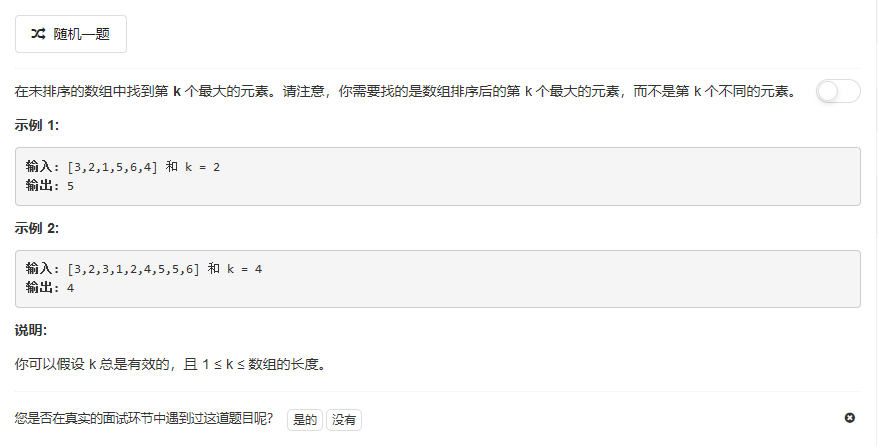public int findKthLargest(int[] nums, int k) {

// 正确性判断
if (0 == nums.length || null == nums || k <= 0 || k > nums.length) {
return -1;
}

// 构造优先队列,默认为最小堆,传入自定义的比较器转换成最大堆
PriorityQueue<Integer> pq = new PriorityQueue<>((a, b) -> b - a);
for (Integer num : nums) {
}
for (int i = 0; i < k - 1; i++) {
pq.remove();
}
return pq.peek();
}

public int findKthLargest(int[] nums, int k) {
if (nums.length == 1) {
return nums;
}

int max = nums;
int min = nums;

for (int i : nums) {
max = i > max ? i : max;
min = i < min ? i : min;
}

int[] arrs = new int[max - min + 1];

for (int i : nums) {
arrs[max - i]++;
}

int pos = 0;
for (int i = 0; i < arrs.length; i++) {
pos += arrs[i];
if (pos >= k) {
return max - i;
}
}

return nums;
}

public int findKthLargest(int[] nums, int k) {
Arrays.sort(nums);
return nums[nums.length - k];
}

## 239. 滑动窗口最大值（类似剑指Offer面试题59）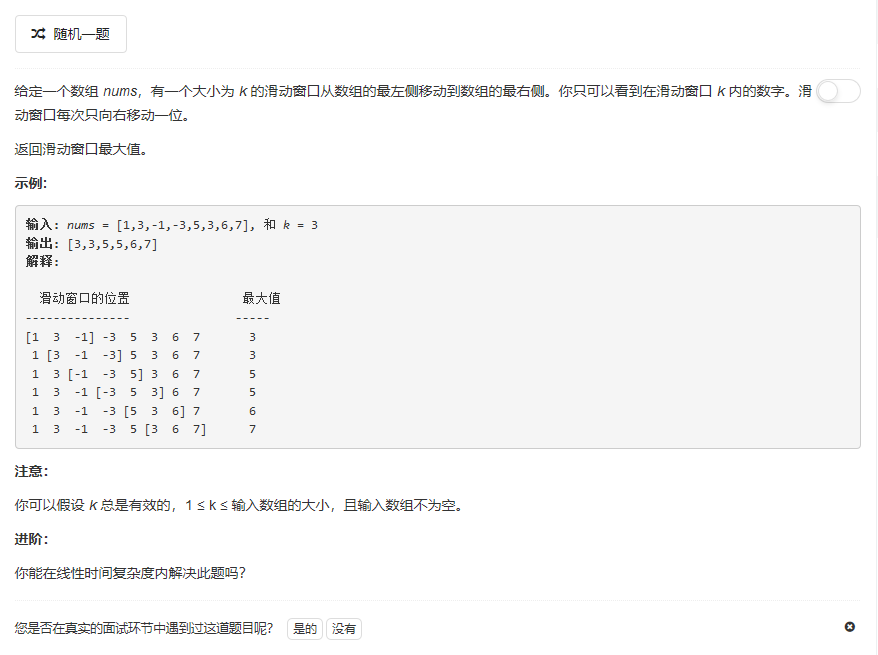public int[] maxSlidingWindow(int[] nums, int k) {
if (nums == null || k <= 0) return new int;
int[] res = new int[nums.length - k + 1];
ArrayDeque<Integer> deque = new ArrayDeque<Integer>();

int index = 0;
for (int i = 0; i < nums.length; i++) {
while (!deque.isEmpty() && deque.peek() < i - k + 1) // Ensure deque's size doesn't exceed k
deque.poll();

// Remove numbers smaller than a[i] from right(a[i-1]) to left, to make the first number in the deque the largest one in the window
while (!deque.isEmpty() && nums[deque.peekLast()] < nums[i])
deque.pollLast();

deque.offer(i);// Offer the current index to the deque's tail

if (i >= k - 1)// Starts recording when i is big enough to make the window has k elements
res[index++] = nums[deque.peek()];
}
return res;
}

public int[] maxSlidingWindow(int[] nums, int k) {
/*

若在当前轮中出现新增的nums[end]大于curMax,直接替换即可；
如果当前轮curMax不是新增的nums[end]，在新的范围内重置curMax.
*/
if (nums.length == 0 || k <= 0)
return new int;
int curMax = Integer.MIN_VALUE;
for (int i = 0; i < k; i++) {
if (nums[i] > curMax)
curMax = nums[i];
}
int[] ans = new int[nums.length - k + 1];
ans = curMax;

for (int start = 1; start + k - 1 < nums.length; start++) {
int end = start + k - 1;
if (nums[end] > curMax)
curMax = nums[end];
else if (nums[start - 1] == curMax) {//新增的不大于curMax，新范围内重置
curMax = Integer.MIN_VALUE;
for (int i = start; i <= end; i++) {
if (nums[i] > curMax)
curMax = nums[i];
}
}
ans[start] = curMax;
}
return ans;
}

## 264. 丑数 II（剑指Offer面试题49）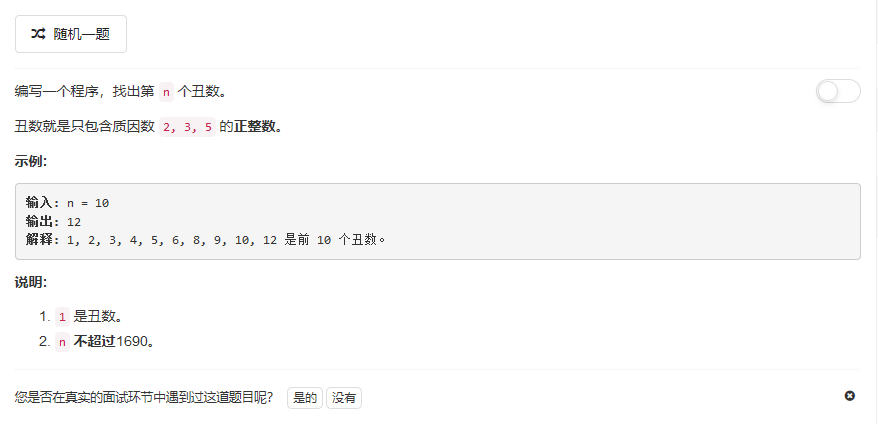public int nthUglyNumber(int n) {
// 正确性判断
if (n < 1 || n > 1690) {
return -1;
}
int[] ugly = new int[n];
ugly = 1;
int index2 = 0, index3 = 0, index5 = 0;
int factor2 = 2, factor3 = 3, factor5 = 5;
for (int i = 1; i < n; i++) {
int min = Math.min(Math.min(factor2, factor3), factor5);
ugly[i] = min;
if (factor2 == min)
factor2 = 2 * ugly[++index2];
if (factor3 == min)
factor3 = 3 * ugly[++index3];
if (factor5 == min)
factor5 = 5 * ugly[++index5];
}
return ugly[n - 1];
}

## 295.数据流的中位数（剑指Offer面试题41）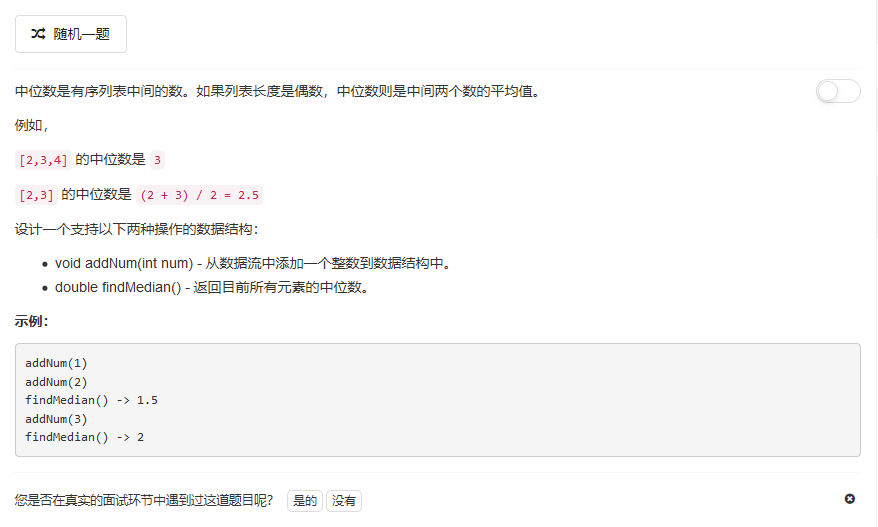public class MedianFinder {

PriorityQueue<Integer> maxHeap;
PriorityQueue<Integer> minHeap;

/**
* initialize your data structure here.
*/
public MedianFinder() {
maxHeap = new PriorityQueue<>(Collections.reverseOrder());
minHeap = new PriorityQueue<>();
}

if (minHeap.size() - maxHeap.size() > 0) {
}
}

public double findMedian() {
if (maxHeap.size() == minHeap.size()) {
return (maxHeap.peek() + minHeap.peek()) / 2.0;
} else {
return maxHeap.peek();
}
}
}

:– | :– | :–

AVL树 | O(logn) | O(logn)

AVL树是一种很高效的数据结构，但是在大多数的语言中都没有现成的实现，所以考虑用最大堆和最小堆，对于一个已经排好序的数据容器，我们可以从中间分开分成两个部分，其中拿P1指向左半部分最大的元素，拿P2指向有半部分最小的元素，如果能够保证数据容器左边的数据都小于右边的数据，那么即使左、右两边内部的数据没有排序，我们仍然可以根据左边最大的数和右边最大的数得到中位数：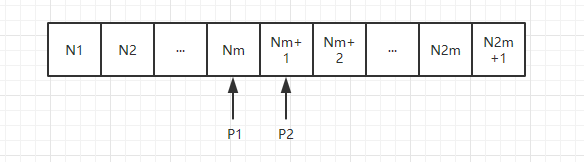## 347. 前K个高频元素（类似剑指Offer面试题40）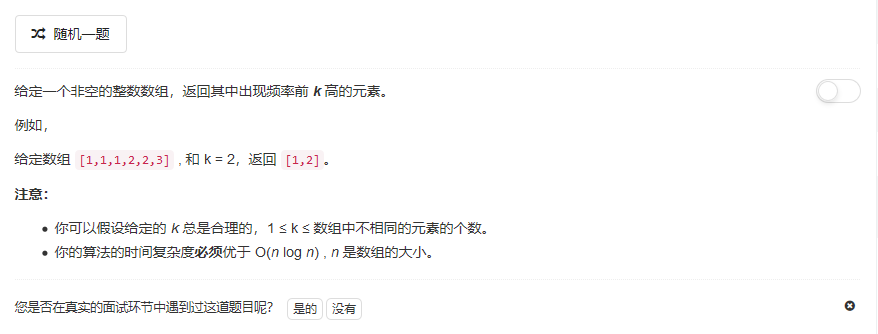public List<Integer> topKFrequent(int[] nums, int k) {
TreeMap<Integer, Integer> map = new TreeMap<>();
// 保存频率
for (int num : nums) {
if (map.containsKey(num)) {
map.put(num, map.get(num) + 1);
} else {
map.put(num, 1);
}
}

PriorityQueue<Integer> pq = new PriorityQueue<>(Comparator.comparingInt(map::get));
for (int key : map.keySet()) {
if (pq.size() < k) {
} else if (map.get(key) > map.get(pq.peek())) {
pq.remove();
}
}

while (!pq.isEmpty()) {
}
return res;
}

## 692. 前K个高频单词public List<String> topKFrequent(String[] words, int k) {
Map<String, Integer> count = new HashMap();
for (String word: words) {
count.put(word, count.getOrDefault(word, 0) + 1);
}
List<String> candidates = new ArrayList(count.keySet());
Collections.sort(candidates, (w1, w2) -> count.get(w1).equals(count.get(w2)) ?
w1.compareTo(w2) : count.get(w2) - count.get(w1));

return candidates.subList(0, k);
}

public List<String> topKFrequent(String[] words, int k) {
Map<String, Integer> count = new HashMap();
for (String word: words) {
count.put(word, count.getOrDefault(word, 0) + 1);
}
PriorityQueue<String> heap = new PriorityQueue<String>(
(w1, w2) -> count.get(w1).equals(count.get(w2)) ?
w2.compareTo(w1) : count.get(w1) - count.get(w2) );

for (String word: count.keySet()) {
heap.offer(word);
if (heap.size() > k) heap.poll();
}

List<String> ans = new ArrayList();
Collections.reverse(ans);
return ans;
}

github：wmyskxz